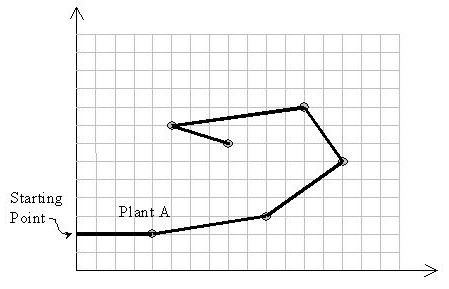# POJ 1696 - Space Ant

## 解题思路

Graham能不用就不用，一代码超长超烦，特别是散点集排序。看过算法理论的同学，一般都宁愿用极坐标而不用水平序，但是极坐标排序的比较规则特容易出错。水平排序我没看懂，估计其他菜鸟们也差不多。## AC 源码

``````//Memory Time
//228K   0MS

#include<iostream>
using namespace std;

const int inf=101;

typedef class
{
public:
int x,y;
}point;

/*AB距离平方*/

int distsquare(point A,point B)
{
return (B.x-A.x)*(B.x-A.x)+(B.y-A.y)*(B.y-A.y);
}

/*叉积计算*/

int det(int x1,int y1,int x2,int y2)
{
return x1*y2-x2*y1;
}

int cross(point A,point B,point C,point D)
{
return det(B.x-A.x , B.y-A.y , D.x-C.x , D.y-C.y);
}

int main(int i)
{
int test;
cin>>test;
while(test--)
{
int n;   //n个点
cin>>n;
point* node=new point[n+1];  //n个点坐标
int* conbag=new int[n+1];    //凸包顶点（根据顶点构造顺序，依次记录node[]下标）
bool* vist=new bool[n+1];    //标记已作为凸包顶点的点

/*Input*/

int min_y=inf;    //最小纵坐标值
int fi=0;
for(i=1;i<=n;i++)
{
int num;
cin>>num>>node[i].x>>node[i].y;
vist[i]=false;

if(min_y > node[i].y)
{
min_y = node[i].y;
fi=i;
}
}
conbag=fi;  //起点
conbag=1;  //凸包顶点计数器
vist[fi]=true;

/*Structure Convex bag*/

int pc=1;   //conbag[]指针
while(true)
{
int s=conbag[pc];  //最新的凸包顶点
int k;    //当前待加入的凸包顶点
for(i=1;i<=n;i++)   //寻找当前基准向量sk,k取任意没标记的点就可以了
if(!vist[i])
{
k=i;
break;
}

for(i=1;i<=n;i++)   //枚举没标记的点i，计算sk X si的值，寻找最优（最外面的）k点作为凸包顶点
if(i!=k && !vist[i])
{
int temp=cross(node[s],node[k],node[s],node[i]);

if(temp<0)
k=i;
else if(temp==0)
if(distsquare(node[s],node[k]) > distsquare(node[s],node[i]))  //当k i共线时，距离s近的点优先选取
k=i;
}

conbag[++pc]=k;   //更新凸包顶点
conbag++;
vist[k]=true;

if(n-conbag==1)   //处理完前面n-1个点后 第n个点不再处理
break;
}

cout<<conbag+1<<' ';  //这里输出n也可以的

fi=0;
for(i=1;i<=conbag;i++)
{
cout<<conbag[i]<<' ';   //输出前面n-1个点的同时寻找第n个没标记的点
if(!vist[i])
fi=i;
}
if(fi)
cout<<fi<<endl;
else
cout<<n<<endl;

delete node;
delete conbag;
delete vist;

}
return 0;
}``````

目录